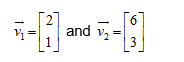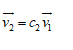Linear Algebra With Applications - 4 Edition - Chapter 3.2 - Problem 10
Register Now

Join StudySoup

Get Full Access to Linear Algebra With Applications - 4 Edition - Chapter 3.2 - Problem 109780136009269

# In Exercises 10 through 20, use paper and pencil to identify the redundant vectors. Thus

Linear Algebra with Applications | 4th Edition

Problem 10

In Exercises 10 through 20, use paper and pencil to identify the redundant vectors. Thus determine whether the given vectors are linearly independent.

Accepted Solution
Step-by-Step Solution:

Step 1 of 2

We want to find out if the given vectors are linearly dependent by finding the redundant vectors. To do this we will let:If one is dependent we can write###### Chapter 3.2, Problem 10 is Solved

Step 2 of 2

Unlock Textbook Solution